• +91 9971497814
• info@interviewmaterial.com

# RD Chapter 18- Binomial Theorem Ex-18.2 Interview Questions Answers

### Related Subjects

Question 1 :

Find the 11th term from the beginning and the 11th termfrom the end in the expansion of (2x – 1/x2)25.

Given:

(2x – 1/x2)25

The given expressioncontains 26 terms.

So, the 11th termfrom the end is the (26 − 11 + 1) th term from thebeginning.

In other words, the 11th termfrom the end is the 16th term from the beginning.

Then,

T16 =T15+1 = 25C15 (2x)25-15 (-1/x2)15

25C15 (210)(x)10 (-1/x30)

= – 25C15 (210 /x20)

Now we shall find the11th term from the beginning.

T11 =T10+1 = 25C10 (2x)25-10 (-1/x2)10

25C10 (215)(x)15 (1/x20)

25C10 (215 /x5)

Question 2 : Find the 7th term in theexpansion of (3x2 – 1/x3)10.

Given:

(3x2 –1/x3)10

Let us consider the 7th termas T7

So,

T7 = T6+1

10C6 (3x2)10-6 (-1/x3)6

10C6 (3)4 (x)8 (1/x18)

= [10×9×8×7×81] /[4×3×2×x10]

= 17010 / x10

The 7th termof the expression (3x2 – 1/x3)10 is17010 / x10.

Question 3 :

Find the 5th term in the expansion of (3x – 1/x2)10.

Given:

(3x – 1/x2)10

The 5th termfrom the end is the (11 – 5 + 1)th, is., 7th term from thebeginning.

So,

T7 = T6+1

10C6 (3x)10-6 (-1/x2)6

10C6 (3)4 (x)4 (1/x12)

= [10×9×8×7×81] /[4×3×2×x8]

= 17010 / x8

The 5th termof the expression (3x – 1/x2)10 is 17010 / x8.

Question 4 :

Find the 8th term in the expansion of (x3/2 y1/2 –x1/2 y3/2)10.

Given:

(x3/2 y1/2 –x1/2 y3/2)10

Let us consider the 8th termas T8

So,

T8 = T7+1

10C7 (x3/2 y1/2)10-7 (-x1/2 y3/2)7

= -[10×9×8]/[3×2] x9/2 y3/2 (x7/2 y21/2)

= -120 x8y12

The 8th termof the expression (x3/2 y1/2 – x1/2 y3/2)10 is-120 x8y12.

Question 5 :

Find the 7th term in the expansion of (4x/5 + 5/2x) 8.

Given:

(4x/5 + 5/2x) 8

Let us consider the 7th termas T7

So,

T7 = T6+1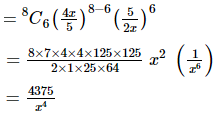The 7th termof the expression (4x/5 + 5/2x) 8 is 4375/x4.

Question 6 :

Find the 4th term from the beginning and 4th termfrom the end in the expansion of (x + 2/x) 9.

Given:

(x + 2/x) 9

Let Tr+1 bethe 4th term from the end.

Then, Tr+1 is(10 − 4 + 1)th, i.e., 7th, term from the beginning.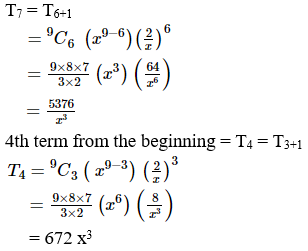Question 7 :

Find the 4th term from the end in the expansion of (4x/5– 5/2x) 9.

Given:

(4x/5 – 5/2x) 9

Let Tr+1 bethe 4th term from the end of the given expression.

Then, Tr+1 is(10 − 4 + 1)th term, i.e., 7th term, from the beginning.

T7 = T6+1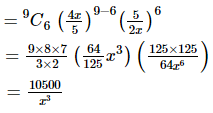The 4th termfrom the end is 10500/x3.

Question 8 :

Find the 7th term from the end in the expansion of (2x2 –3/2x) 8.

Given:

(2x2 –3/2x) 8

Let Tr+1 bethe 4th term from the end of the given expression.

Then, Tr+1 is(9 − 7 + 1)th term, i.e., 3rd term, from the beginning.

T3 = T2+1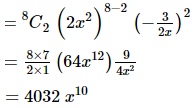The 7th termfrom the end is 4032 x10.

Question 9 :

Find the coefficient of:

(i)  x10 in the expansion of (2x2 –1/x)20

(ii) x7 in the expansion of (x – 1/x2)40

(iii) x-15 in the expansion of (3x2 –a/3x3)10

(iv) x9 in the expansion of (x2 – 1/3x)9

(v) xm in the expansion of (x + 1/x)n

(vi) x in the expansion of (1 – 2x3 + 3x5) (1+ 1/x)8

(vii) a5b7 in the expansion of (a – 2b)12

(viii) x in the expansion of (1 – 3x + 7x2) (1 – x)16

(i)  x10 inthe expansion of (2x2 – 1/x)20

Given:

(2x2 –1/x)20

If  x10 occursin the (r + 1)th term in the given expression.

Then, we have:

Tr+1 nCr xn-r ar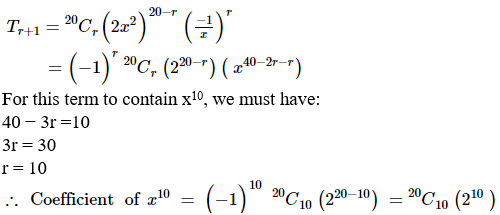(ii) x7 inthe expansion of (x – 1/x2)40

Given:

(x – 1/x2)40

If xoccursat the (r + 1) th term in the given expression.

Then, we have:

Tr+1 nCr xn-r ar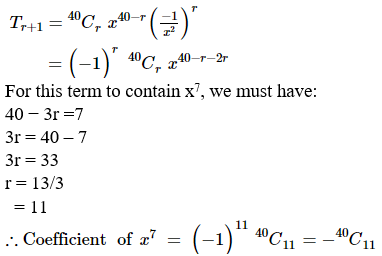40 − 3r =7

3r = 40 – 7

3r = 33

r = 33/3

= 11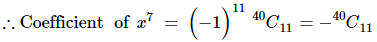(iii) x-15 inthe expansion of (3x2 – a/3x3)10

Given:

(3x2 –a/3x3)10

If x−15 occursat the (r + 1)th term in the given expression.

Then, we have:

Tr+1 nCr xn-r ar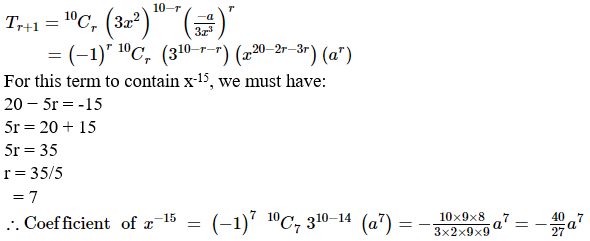(iv) x9 inthe expansion of (x2 – 1/3x)9

Given:

(x2 –1/3x)9

If x9 occursat the (r + 1)th term in the above expression.

Then, we have:

Tr+1 nCr xn-r ar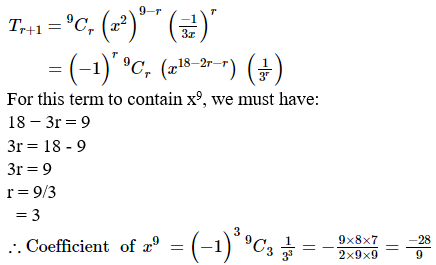For this term to contain x9, we must have:

18 − 3r = 9

3r = 18 – 9

3r = 9

r = 9/3

= 3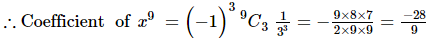(v) xm inthe expansion of (x + 1/x)n

Given:

(x + 1/x)n

If xm occursat the (r + 1)th term in the given expression.

Then, we have:

Tr+1 nCr xn-r ar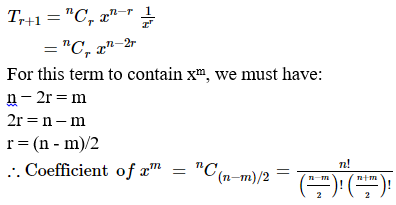(vi) x in the expansion of(1 – 2x3 + 3x5) (1 + 1/x)8

Given:

(1 – 2x3 +3x5) (1 + 1/x)8

If x occursat the (r + 1)th term in the given expression.

Then, we have:

(1 – 2x3 +3x5) (1 + 1/x)8 = (1– 2x3 + 3x5) (8C0 + 8C1 (1/x)+ 8C2 (1/x)2 + 8C3 (1/x)3 + 8C4 (1/x)4 + 8C5 (1/x)5 + 8C6 (1/x)6 + 8C7 (1/x)7 + 8C8 (1/x)8)

So, ‘x’ occurs in theabove expression at -2x3.8C2 (1/x2)+ 3x5.8C4 (1/x4)

Coefficient of x = -2(8!/(2!6!)) + 3 (8!/(4! 4!))

= -56 + 210

= 154

(vii) a5b7 inthe expansion of (a – 2b)12

Given:

(a – 2b)12

If a5b7 occursat the (r + 1)th term in the given expression.

Then, we have:

Tr+1 nCr xn-r ar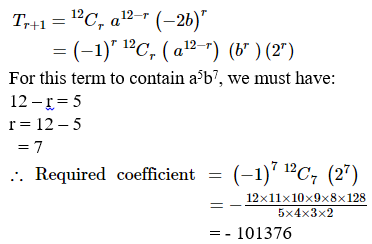(viii) x in the expansion of(1 – 3x + 7x2) (1 – x)16

Given:

(1 – 3x + 7x2)(1 – x)16

If x occursat the (r + 1)th term in the given expression.

Then, we have:

(1 – 3x + 7x2)(1 – x)16 = (1 – 3x + 7x2) (16C0 + 16C1 (-x)+ 16C2 (-x)2 + 16C3 (-x)3 + 16C4 (-x)4 + 16C5 (-x)5 + 16C6 (-x)6 + 16C7 (-x)7 + 16C8 (-x)8 + 16C9 (-x)9 + 16C10 (-x)10 + 16C11 (-x)11 + 16C12 (-x)12 + 16C13 (-x)13 + 16C14 (-x)14 + 16C15 (-x)15 + 16C16 (-x)16)

So, ‘x’ occurs in theabove expression at 16C1 (-x) – 3x16C0

Coefficient of x =-(16!/(1! 15!)) – 3(16!/(0! 16!))

= -16 – 3

= -19

Question 10 : Which term in the expansion of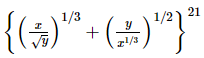contains x and y to one and the same power.

Let us consider Tr+1 thterm in the given expansion contains x and y to one and the same power.

Then we have,

Tr+1 = nCr xn-r ar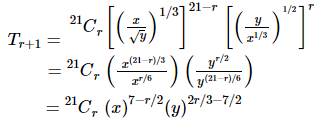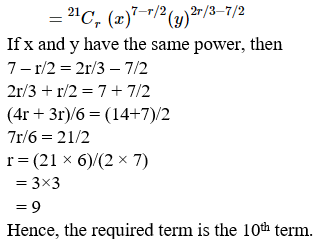Todays Deals### RD Chapter 18- Binomial Theorem Ex-18.2 Contributorskrishan

Name:
Email:

# Latest News# 9000 interview questions in different categories